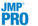Publication date: 06/16/2020

##Data Processing Report Options

The Data Processing red triangle menu contains the following options:

Cleanup

A submenu of the following data cleanup options:

Remove Zeros

Removes observations with zero values. If there are no zeros in the data, an alert appears, indicating that no zero values were found.

Remove Value

Displays a specifications window that enables you to specify a value to remove from the data.

Remove Selected

Removes observations that correspond to rows that are selected in the data table.

Remove Unselected

Removes observations that correspond to rows that are not selected in the data table.

Filter X

Removes X, Input values that fall outside of a specified interval. When you select the Filter X option, you must specify Below and Above values. The X, Input values that fall outside of the specified interval are not used for the analysis.

Filter Y

Removes Y, Output values that fall outside of a specified interval. When you select the Filter Y option, you must specify Below and Above values. The Y, Output values that fall outside of the specified interval are not used for the analysis.

Note: The remove options exclude the specified observations from the analysis report, but the observations remain unchanged in the data table.

Transform

A submenu of the following options to transform the output data:

Center

Centers the output.

Standardize

Standardizes the output by centering and scaling the data to have mean 0 and variance 1.

Range 0 to 1

Scales the output to lie within the range of 0 and 1.

Square Root

Transforms the data by computing the square root of the output. The output values must be nonnegative.

Square

Transforms the data by computing the square of the output.

Log

Transforms the data by computing the natural logarithm of the output.

Exp

Transforms the data by computing the exponential function of the output.

Negation

Transforms the data by negating the output.

Logit

Transforms the data by computing the logit function of the output. The output values must be between 0 and 1.

Align

A submenu of the following options to align the input data:

Row Alignment

Replaces the input values with the row number.

Align Maximum

Aligns the functions using the observed maximum output value for each ID level. The input value associated with the observed maximum output value is set to zero for each ID level and the other input values are shifted up or down based on the difference between the observed maximum and zero.

Align Minimum

Aligns the functions using the observed minimum output value for each ID level. The input value associated with the observed minimum output value is set to zero for each ID level and the other input values are shifted up or down based on the difference between the observed minimum and zero.

Align Range 0 to 1

Aligns the output functions such that the range of the input values is 0 to 1.

Tip: Align Range 0 to 1 is particularly useful when you fit a P-Spline model.

Dynamic Time Warping

(Available only when there is more than one function.) Aligns the output functions using dynamic time warping (DTW). DTW is a function alignment technique that finds an optimal warping to align two or more functions together. When you select the DTW option, a Select Reference Function window appears. Use this to select the reference function. The reference function is the function that the remaining functions are aligned to.

Once you select a reference function and click OK, a warping function plot is shown along with a list for the remaining query functions. On the warping function plot, the reference function is on the y-axis and the selected query function is on the x-axis. Deviations from the red diagonal line (y = x) indicate that the inputs of the query function have been warped for better alignment.

Target Functions

(Available only when there is more than one function.) A submenu that enables you to load target functions.

Shows a window that enables you to specify a target function. A target function is used for curve matching, where it is desirable for all of the functions to look like the target function. You can also specify two target functions to compare the remaining curves to the “best” and “worse” case functions.

If you specify one or more target functions, the data from the functions are not used in model fitting. For each specified target function, two rows are added to the FPC Profiler. See FPC Profiler.

### Dynamic Time Warping Options

Plot Warping Functions

Shows or hides the warping function plot. On by default.

Save Distance Matrix

Saves the distance matrix to a separate data table. The distance matrix can be useful for clustering the functions. The distance matrix data table contains a hierarchical clustering script.

Save Warping Functions

Saves the warping functions to a separate data table. Each row of the data table contains the DTW adjusted input variable, the original input variable, and the ID variable.